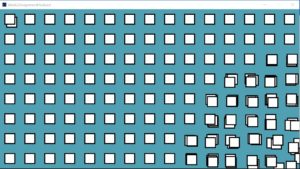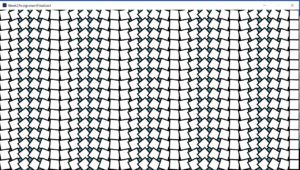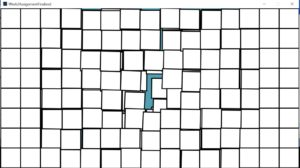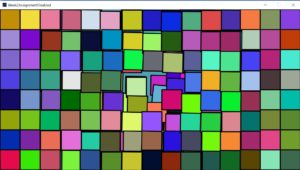# Week 2: Draw a Computer Graphic using Loops

#### Introduction:

`As for the week 2 assignment, I must explore more features of processing.org. The hardcoded way of drawing sketches and graphics is time consuming and takes a lot of computer memory. In this assignment, I got the opportunity to explore how to repeat the functionalities to enhance the reusability of code. Furthermore, I explored some new mathematical functions which helped me put some sort of randomization in my graphics. This assignment not only aided in building my logic behind computer graphics, but also how to visualize a genuine look for the shapes.`

#### The inspiration behind computer graphic image:

`I should draw an old era computer magazines computer graphic via processing. The computer graphic I selected was from Computer Graphics & Art. The main point that drew me into it is its imperfection. The squares are not in perfect order, but it is still colorful and beautiful. It looks pleasing to the eye and its main attraction is its chaotic imperfection. In short, it represents a beautiful yet chaotic work of art. It does not have to be perfect to be beautiful.`

#### Challenges and Problems:

`The computer graphic I selected is simple and consistent during visualization on screen but building the back end logic is challenging on its own. I faced issues while attaching each brick to the right place with some movement either in a horizontal or vertical direction which makes the center of image looks like its breaking apart. It was challenging for me to move the square shapes in random directions with random rotation. I worked more on the center of the wall as most of the random movement and rotation of bricks was concentrated at the center. Not only was giving more randomization at the center a challenging task, but also providing less randomization at the corners to give the computer graphic image a sense that it is breaking from the center was not easy either. At a point when I was using loops, I got stuck. Running the code while trying to move the shapes for infinite times made it hard for me to organize the code in a reusable pattern.`

#### Procedure:

`First, I read out the official processing built-in functions and their use to try and learn them better. In the very first step, I compiled a list of built-in functions which I thought would be useful later in my code. I took note of those built-in functions signature and parameter for further usage. The most frequent used function is the random () function. However, randomization always requires some initial value. I sketched out the logic and began sketching the graphic from the screen central point. I initialized each brick size and then used each brick central point to place every other brick next to each other. I placed all the bricks next to each other in vertical and horizontal directions by using two loops which iterates continuously in horizontal and vertical directions. This is ongoing until the bricks fill up the whole screen. The whole grid was constructed by the nested for () loop. During the placement of each brick, Brick Position () function is calculating how far the brick is from the screen center. Thus, based on that gap, the rotation and movement of brick is calculated by using the dist () function. The parameters I gave to dist() function was the position of the brick and screen central positions x and y coordinates. Later, I normalized the gap in the bricks, where if the gap is large, then random rotation and movement will be less.`
``` //Global Varible for Screen Width and height
float ScreenH,ScreenW;

//Global Varible for the size of one brick.
//Assuming width and height of each brick is same
int SizeOfBrick;

//Gobal Variable to calculate how much space between two bricks
float InterSpaceLengthScale;

//To find out Space between Brick and Centeral Screen Area
float BrickPosition(int brickx,int bricky)
{
return dist(brickx,bricky, ScreenW/2, ScreenH/2);
}```
`This is achieved by multiplying the random values with the space normalized gap that is between each brick and screen central area. Meanwhile, to give each brick a random color scheme, I picked a random value of red, green, and blue from 0 to 255. Then, when each brick is placed on the right place with the right movement and rotation, I fill the brick with a random RGB value using fill () function.`
```void setup() {

//Initializing the screen of given demensions
size(1500, 800);
strokeWeight(5);
ScreenW=width;
ScreenH=height;

SizeOfBrick = 100;
InterSpaceLengthScale=SizeOfBrick;

// Changing the origin of rectangle shape towards center
rectMode(CENTER);

background(#519FB2);

DrawBricksGrid();
}```
`What was interesting to learn was that I can use functions inside any function, unless the function is used as a parameter return value in a required data type. I found out that processing built-in draw () function and for() loop works in the same way. The only difference was that draw () function works for infinite time with non-termination, while in for () loop we give it a termination condition. So, the internal for () loop terminates when the height of screen fills up while the outer for() loop terminates when the screen width fills up.`

#### Bricks Movement:

`Inside the nested for loop (), I gave a random movement and rotation to each new brick using translate () and rotation() function. The translate () function moves the brick to a random position from its original position. In the image below, I used Brick Movement() function which is returning some random new position based on the original position of the brick and the gap from the screen center.`
```//Calculating InterSpace Scale.
void BrickSpan(float InterSpaceLength)
{
if (InterSpaceLength > SizeOfBrick*6)
{
InterSpaceLengthScale = InterSpaceLengthScale * .01;
}
else
{
InterSpaceLengthScale= 1/InterSpaceLength * 100;
}
}

//Bricks Movement in 2D place along X and Y axis
float BrickMovement()
{
return  random(50) * InterSpaceLengthScale;
}
```#### Bricks rotation:

`Each brick is rotating to some angle which is calculated based on the original brick position and central screen position. I implemented BrickRotation() function which returns a random angle value by multiplying the new random angle with the normalized gap between brick original position and screen central point. As I drew the graphic on a 2D plane, I made use of pop Matrix() and pushMatrix() when drawing each brick to its current and previous state of the 2D plane coordinate system. The random transformation applies to each and every brick inside the nested for() function.`
```//Rotation of Brick
float BrickRotation()
{
return random(PI/60) * InterSpaceLengthScale;
}

void DrawBricksGrid()
{
for (int i=SizeOfBrick/2; i<ScreenW; i=i+SizeOfBrick)
{

for (int j=SizeOfBrick/2; j<ScreenH; j=j+SizeOfBrick)
{

float gap = BrickPosition(i,j);
BrickSpan(gap);

float r = random(256);
float g = random(256);
float b = random(256);
fill(r,g,b);
pushMatrix();

translate(i+BrickMovement(), j+BrickMovement());
rotate(BrickRotation());

rect(0, 0, SizeOfBrick, SizeOfBrick);
popMatrix();
}
}
}
```#### Final Artwork Images :#### Conclusion:

`In this assignment, I understood the proper use of for() loops to draw shapes multiple times, how to apply transformation of shapes, how to move objects from one position to another, as well as object rotation. I can better organize my code and enhance its reusability by implementing custom functions. Moreover, I got the knowledge on how to apply randomization in function calling, and on how to draw shapes to give the art piece a consistent look.Thus, I learned how to work on what you see is what you get pattern by calling custom implemented functions and using built in functions. Finally, I can also reserve the 2D plane coordinate system state by using popMatrix() and pushMatrix() functions.`

#### Full Code Packed All Together:

```//Global Varible for Screen Width and height
float ScreenH,ScreenW;

//Global Varible for the size of one brick.
//Assuming width and height of each brick is same
int SizeOfBrick;

//Gobal Variable to calculate how much space between two bricks
float InterSpaceLengthScale;

//To find out Space between Brick and Centeral Screen Area
float BrickPosition(int brickx,int bricky)
{
return dist(brickx,bricky, ScreenW/2, ScreenH/2);
}

//Calculating InterSpace Scale.
void BrickSpan(float InterSpaceLength)
{
if (InterSpaceLength > SizeOfBrick*6)
{
InterSpaceLengthScale = InterSpaceLengthScale * .01;
}
else
{
InterSpaceLengthScale= 1/InterSpaceLength * 100;
}
}

//Bricks Movement in 2D place along X and Y axis
float BrickMovement()
{
return  random(50) * InterSpaceLengthScale;
}

//Rotation of Brick
float BrickRotation()
{
return random(PI/60) * InterSpaceLengthScale;
}

void DrawBricksGrid()
{
for (int i=SizeOfBrick/2; i<ScreenW; i=i+SizeOfBrick)
{

for (int j=SizeOfBrick/2; j<ScreenH; j=j+SizeOfBrick)
{

float gap = BrickPosition(i,j);
BrickSpan(gap);

float r = random(256);
float g = random(256);
float b = random(256);
fill(r,g,b);
pushMatrix();

translate(i+BrickMovement(), j+BrickMovement());
rotate(BrickRotation());

rect(0, 0, SizeOfBrick, SizeOfBrick);
popMatrix();
}
}
}

void setup() {

//Initializing the screen of given demensions
size(1500, 800);
strokeWeight(5);
ScreenW=width;
ScreenH=height;

SizeOfBrick = 100;
InterSpaceLengthScale=SizeOfBrick;

// Changing the origin of rectangle shape towards center
rectMode(CENTER);

background(#519FB2);

}

void draw()
{
frameRate(1);
DrawBricksGrid();

}```